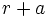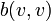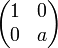# Orthogonal group for a symmetric bilinear form

This term associates to every field, a corresponding group property. In other words, given a field, every group either has the property with respect to that field or does not have the property with respect to that field

This group property is natural number-parametrized, in other words, for every natural number, we get a corresponding group property

## Definition

Let$K$ be a field (of characteristic not equal to two) and$V$ be a vector space over$K$. Consider a symmetric bilinear form$b: V \times V \to K$. Then, the orthogonal group for$b$, denoted as$O(b,k)$, is defined as the group of all invertible linear transformations$A$ such that:$\! b(v,w) = b(Av,Aw)$

for all$v,w \in V$.

Note that sometimes, we use the term orthogonal space for the space$V$ equipped with the form$b$.

Although this definition makes sense for an arbitrary symmetric bilinear form, we typically assume that the bilinear form is nondegenerate, in the sense that there is no nonzero vector$v \in V$ such that$b(v,w) = 0$ for all$w \in V$.

The two main examples for a finite-dimensional vector space are the orthogonal group for the standard dot product and the split orthogonal group.

## Classification

The classification of orthogonal groups over fields of characteristic not equal to two proceeds based on the classification of symmetric bilinear forms up to equivalence. The basic result in that classification is the following decomposition theorem based on the work of Witt:

The classification thus reduces to classifying anisotropic spaces (or equivalently, anisotropic forms).

### Over complex numbers (and other algebraically closed fields)

Over complex numbers, and over any algebraically closed field, any two symmetric bilinear forms are equivalent. Hence, the pseudo-orthogonal groups corresponding to symmetric bilinear forms are all conjugate to the standard orthogonal group.

Interpretation in terms of the decomposition theorem: Over the complex numbers, and in general over all algebraically closed fields, the maximum possible dimension of a space with an anisotropic form is$1$, and this space is uniquely determined up to isometry. Thus, if the total dimension of the vector space is odd, it is the direct sum of a hyperbolic space and an anisotropic space, while if the total dimension is even, it is a hyperbolic space.

### Over real numbers

Over real numbers, and over any real-closed field, there are$n+1$ types of pseudo-orthogonal groups for vector spaces of dimension$n$. For each of these, we can choose as the bilinear form, one of the signature matrices (viz., a diagonal matrix with some 1s and some -1s).

Note that the pseudo-orthogonal groups for inequivalent bilinear forms may be isomorphic. For instance, the pseudo-orthogonal group for$b$ and$-b$ are isomorphic, even though these forms are in general not equivalent -- they have signatures that are opposites of each other.

Interpretation in terms of the decomposition theorem: Since there exist positive definite forms, there is no bound on the dimension of the anisotropic subspace. If the orthogonal space is a sum of a maximal hyperbolic space of dimension$2r$ and an anisotropic space of dimension$a$, then the signature matrix has$r + a$ 1s and$r$ -1s or$r$ 1s and$r + a$ -1s.

### Over finite fields

The discussion below combines ideas from every orthogonal space is a direct sum of a hyperbolic and an anisotropic space and classification of anisotropic spaces over finite fields.

Over a finite field of characteristic not equal to two, there are the following two cases:

Parity of dimension of vector space$q$ mod 4 Number of equivalence classes of symmetric bilinear forms Number of orthogonal groups Explanation Where does the standard dot product fall?
odd 1 or 3 2 1 Both bilinear forms are of the following type: orthogonal direct sum of a hyperbolic space of codimension one and a one-dimensional space with generator$v$. In one bilinear form, the image$b(v,v)$ is a square and in the other, it is a non-square.
Both have isomorphic orthogonal groups.
even 1 2 2 There is one bilinear form that is hyperbolic, i.e., the vector space decomposes as a direct sum of hyperbolic planes. There is also another bilinear form where the vector space is the orthogonal direct sum of a hyperbolic subspace of codimension two and a plane on which the form is equivalent to$\begin{pmatrix} 1 & 0 \\ 0 & a \\\end{pmatrix}$ where$a$ is a quadratic nonresidue.
even 3 2 2 There is one bilinear form that is hyperbolic, i.e., the vector space decomposes as a direct sum of hyperbolic planes. There is also another bilinear form where the vector space is the orthogonal direct sum of a hyperbolic subspace of codimension two and a plane on which the form is equivalent to$\begin{pmatrix} 1 & 0 \\ 0 & 1 \\\end{pmatrix}$ the hyperbolic + anisotropic case